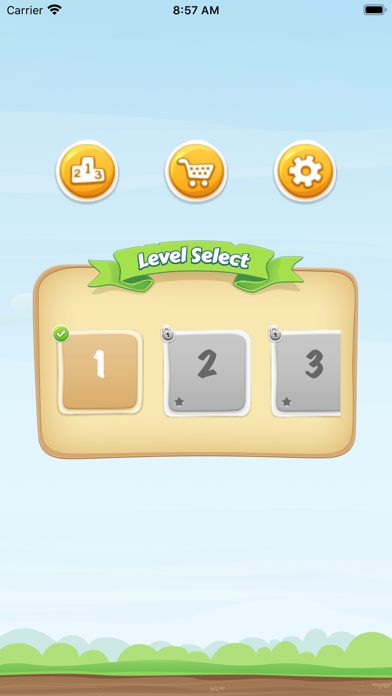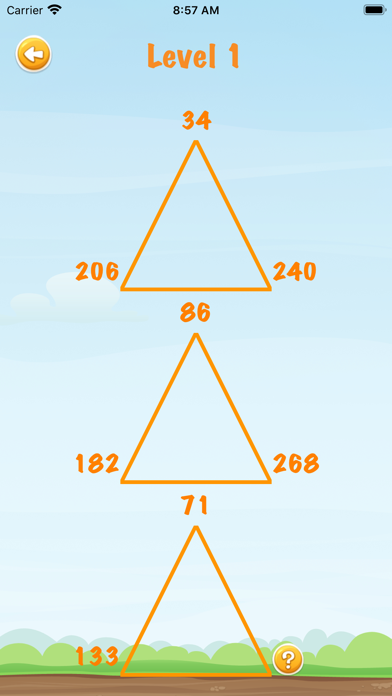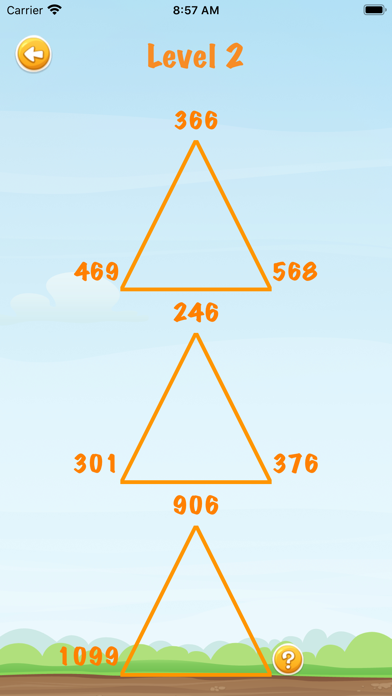# Super Brain Test Math IQ

## iOS UniversalGames

Free

Do you like math?
Do you like solving algorithms?
IF SO you should try Brain Out :IQ Challenge Math ,it made me smarter and if it can make me smarter it can make YOU smarter The best thing about Brain Out :IQ Challenge Math is that you get to solve fun algorithms and do fun math problems and multiplication and addition and subtraction and division it teaches you math tricks and other kinds of stuff I need practice on my multiplication . You should try this NOW!!

== HOW TO USE ==
• Choose a LEVEL when you need concentrate quick

• Thinking and looking for the logic of test

Example:
Triangle1: 57,63,120
Triangle2: 82,63,145
Triangle3: 107,20,?
? = 127
Because of:
120 = 57 + 63
145 = 82 + 63
127 = 107+20
• There are total 12 levels from easy, medium, hard to insane

Good luck and enjoy

•••